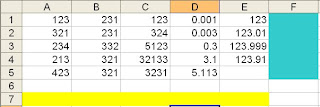## Pages

### Thursday-Friday, May 28-29, 2009

Objective(s):
1. Students will take a MIDTERM EXAM for the EXCEL unit.
2. Students will complete and graph their DATA from the senses experiment.

Agenda:
1. Digital Zen
2. Announcements and Quick Review
3. Midterm (Open-Notes)
4. Complete and Graph Data from senses experiment
5. Graphing Analysis and Stamp
6. Notebook Check (If time)

Digital Zen:

1. If I wanted to multiply A3 with C3, what formula would I type?
2. Write the formula for 20 divided by 5
3. Which is the correct formula for adding a Column: =SUM(A3:A12) or =(A3:A12)?
4. When should you use the word "SUM" in your formula?
5. If tax is 10.25%, write a formula that would figure out the tax for a subtotal of \$23.95 that is in CELL D16?
6. What company makes EXCEL?
8. On Excel, make a quick Reciept for these 2 items. YOU DON'T NEED TO SHOW THIS TO ME FOR CREDIT, just write your answers on BLOGGER. 1 Box of Tissues (\$1.59 EACH) and 2 Toothbrushes (\$2.59 Each). Figure out SUBTOTAL, TAX (9.25%), and GRANDTOTAL.
- What is the SUBTOTAL?
- What is the TAX?
- What is the GRANDTOTAL?

QUIZ FIGURES:

CLICK on EACH SHEET TO ZOOM IN:

SHEET #1:SHEET #2: